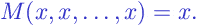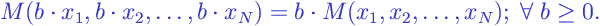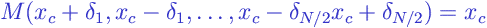# Central Tendency (Measures)

Central Tendency (Measures):

Any measure of central tendency provides a typical value of a set ofvalues. Normally, it is a value around which valuesare grouped. The most widely used measures of central tendency are (arithmetic) mean , median , trimmed mean , mode . Measures of central tendency are defined for a population and for a sample .

For example, two samples – (8,9,10,11,12) and (18,19,20,21,22) have central locations differing by 10 units, and most measures of central location would give values 10 and 20 of the two samples, respectively.

Measuresof central tendency normally meet the following requirements:

• If all valuescoincide – i.e. all are equal to the same value– then the measureis equal to, or formally• The valueis within the interval between the minimal and the maximal value of the set:• Measurehas a property of shift invariance :wheremay be negative.

• Measurehas a property of scale invariance :Most measures of central tendency also have the following property: If the set of valuesis symmetrical with respect to a value(the center), then the value of the measurecoincides with the center. “Symmetrical” here means that, for each valuedifferent from, there is another valuedeviating from the centerby the same magnitude as, but in the opposite direction. (More generally, “Symmetrical” means that the right and left sides of a distribution look the same, in mirror image.)Note that some measures are often classified as measures of central tendency (and have “mean” in their names) but do not meet the requirement of shift invariance. Such measures are usually defined mathematically only for non-negative valuesand, practically, are applicable to quantities that are non-negative in principle – e.g. price, time or space interval, weight, etc. Strictly speaking, such descriptive statistics measure “effective magnitude” or “average magnitude” rather than central tendency. Some examples of such measures are: the power mean , the harmonic mean , the geometric mean , root mean square .

Browse Other Glossary Entries

## Test Yourself

Planning on taking an introductory statistics course, but not sure if you need to start at the beginning? Review the course description for each of our introductory statistics courses and estimate which best matches your level, then take the self test for that course. If you get all or almost all the questions correct, move on and take the next test.

### Data Analytics

Considering becoming adata scientist, customer analyst or our data science certificate program?

Analytics Quiz

Statistics Quiz

### Statistics

Looking at statistics for graduate programs or to enhance your foundational knowledge?

Statistics 1 Quiz

Regression Quiz

Regression Quiz

### Biostatistics

Entering the biostatistics field? Test your skill here.

Biostatistics Quiz

Statistics 2 Quiz

### Stay Informed

Our Blog

Read up on our latest blogs

Certificates

Courses

Find the right course for you